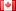# Revision history [back]

### simplify coefficients of laurent series?

I am working with a Laurent series f. My goal is to calculate the principal part of f^3. The code I am using is this:

R. = LaurentSeriesRing(SR, 'u'); R b2, b1, a0, a1, a2, a3, a4 = var('b2 b1 a0 a1 a2 a3 a4') f = b2u^-2 + b1u^-1 + a0 + a1u + a2u^2 + a3u^3 + a4u^4 + O(u^5) f^3

b2^3u^-6 + 3b1b2^2u^-5 + (a0b2^2 + 2b1^2b2 + (2a0b2 + b1^2)b2)u^-4 + (2a0b1b2 + a1b2^2 + (2a0b2 + b1^2)b1 + 2(a0b1 + a1b2)b2)u^-3 + (2a1b1b2 + a2b2^2 + (2a0b2 + b1^2)a0 + 2(a0b1 + a1b2)b1 + (a0^2 + 2a1b1 + 2a2b2)b2)u^-2 + (2a2b1b2 + a3b2^2 + (2a0b2 + b1^2)a1 + 2(a0b1 + a1b2)a0 + 2(a0a1 + a2b1 + a3b2)b2 + (a0^2 + 2a1b1 + 2a2b2)b1)u^-1 + (2a3b1b2 + a4b2^2 + (2a0b2 + b1^2)a2 + 2(a0b1 + a1b2)a1 + 2(a0a1 + a2b1 + a3b2)b1 + (a0^2 + 2a1b1 + 2a2b2)a0 + (2a0a2 + a1^2 + 2a3b1 + 2a4b2)b2) + O(u)

SAGE does not simplify the coefficients of the result. Is there some command that I can enter that will allow me to simultaneously simplify the coefficients of each power of u and output the result up to O(u)? The simplify command does not seem to work.

### simplify coefficients of laurent series?

I am working with a Laurent series f. My goal is to calculate the principal part of f^3. The code I am using is this:

R. = LaurentSeriesRing(SR, 'u'); R R

b2, b1, a0, a1, a2, a3, a4 = var('b2 b1 a0 a1 a2 a3 a4') a4')

f = b2u^-2 + b1u^-1 + a0 + a1u + a2u^2 + a3u^3 + a4u^4 + O(u^5) O(u^5)

f^3

b2^3u^-6 + 3b1b2^2u^-5 + (a0b2^2 + 2b1^2b2 + (2a0b2 + b1^2)b2)u^-4 + (2a0b1b2 + a1b2^2 + (2a0b2 + b1^2)b1 + 2(a0b1 + a1b2)b2)u^-3 + (2a1b1b2 + a2b2^2 + (2a0b2 + b1^2)a0 + 2(a0b1 + a1b2)b1 + (a0^2 + 2a1b1 + 2a2b2)b2)u^-2 + (2a2b1b2 + a3b2^2 + (2a0b2 + b1^2)a1 + 2(a0b1 + a1b2)a0 + 2(a0a1 + a2b1 + a3b2)b2 + (a0^2 + 2a1b1 + 2a2b2)b1)u^-1 + (2a3b1b2 + a4b2^2 + (2a0b2 + b1^2)a2 + 2(a0b1 + a1b2)a1 + 2(a0a1 + a2b1 + a3b2)b1 + (a0^2 + 2a1b1 + 2a2b2)a0 + (2a0a2 + a1^2 + 2a3b1 + 2a4b2)b2) + O(u)

SAGE does not simplify the coefficients of the result. Is there some command that I can enter that will allow me to simultaneously simplify the coefficients of each power of u and output the result up to O(u)? The simplify command does not seem to work.3 No.3 RevisionDSM5242 ●21 ●68 ●112

### simplify coefficients of laurent series?

I am working with a Laurent series f. My goal is to calculate the principal part of f^3. The code I am using is this:

R.

R.<u> = LaurentSeriesRing(SR, 'u'); R R

b2, b1, a0, a1, a2, a3, a4 = var('b2 b1 a0 a1 a2 a3 a4') a4')

f = b2u^-2 + b1u^-1 b2*u^-2 + b1*u^-1 + a0 + a1u + a2u^2 + a3u^3 + a4u^4 + O(u^5) f^3a1*u + a2*u^2 + a3*u^3 + a4*u^4 + O(u^5)

f^3


b2^3u^-6 + 3b1b2^2u^-5 + (a0b2^2 + 2b1^2b2 + (2a0b2 + b1^2)b2)u^-4 + (2a0b1b2 + a1b2^2 + (2a0b2 + b1^2)b1 + 2(a0b1 + a1b2)b2)u^-3 + (2a1b1b2 + a2b2^2 + (2a0b2 + b1^2)a0 + 2(a0b1 + a1b2)b1

b2^3*u^-6 + 3*b1*b2^2*u^-5 + (a0*b2^2 + 2*b1^2*b2 + (2*a0*b2 + b1^2)*b2)*u^-4 + (2*a0*b1*b2 + a1*b2^2 + (2*a0*b2 + b1^2)*b1 + 2*(a0*b1 + a1*b2)*b2)*u^-3 + (2*a1*b1*b2 + a2*b2^2 + (2*a0*b2 + b1^2)*a0 + 2*(a0*b1 + a1*b2)*b1 + (a0^2 + 2a1b1 + 2a2b2)b2)u^-2 + (2a2b1b2 + a3b2^2 + (2a0b2 + b1^2)a1 + 2(a0b1 + a1b2)a0 + 2(a0a1 + a2b1 + a3b2)b2 2*a1*b1 + 2*a2*b2)*b2)*u^-2 + (2*a2*b1*b2 + a3*b2^2 + (2*a0*b2 + b1^2)*a1 + 2*(a0*b1 + a1*b2)*a0 + 2*(a0*a1 + a2*b1 + a3*b2)*b2 + (a0^2 + 2a1b1 + 2a2b2)b1)u^-1 + (2a3b1b2 + a4b2^2 + (2a0b2 + b1^2)a2 + 2(a0b1 + a1b2)a1 + 2(a0a1 + a2b1 + a3b2)b1 2*a1*b1 + 2*a2*b2)*b1)*u^-1 + (2*a3*b1*b2 + a4*b2^2 + (2*a0*b2 + b1^2)*a2 + 2*(a0*b1 + a1*b2)*a1 + 2*(a0*a1 + a2*b1 + a3*b2)*b1 + (a0^2 + 2a1b1 + 2a2b2)a0 + (2a0a2 2*a1*b1 + 2*a2*b2)*a0 + (2*a0*a2 + a1^2 + 2a3b1 + 2a4b2)b2) + O(u)2*a3*b1 + 2*a4*b2)*b2) + O(u)


SAGE does not simplify the coefficients of the result. Is there some command that I can enter that will allow me to simultaneously simplify the coefficients of each power of u and output the result up to O(u)? The simplify command does not seem to work.4 retaggedFrédéricC4383 ●3 ●37 ●93

### simplify coefficients of laurent series?

I am working with a Laurent series f. My goal is to calculate the principal part of f^3. The code I am using is this:

R.<u> = LaurentSeriesRing(SR, 'u'); R

b2, b1, a0, a1, a2, a3, a4 = var('b2 b1 a0 a1 a2 a3 a4')

f = b2*u^-2 + b1*u^-1 + a0 + a1*u + a2*u^2 + a3*u^3 + a4*u^4 + O(u^5)

f^3


b2^3*u^-6 + 3*b1*b2^2*u^-5 + (a0*b2^2 + 2*b1^2*b2 + (2*a0*b2 + b1^2)*b2)*u^-4 + (2*a0*b1*b2 + a1*b2^2 + (2*a0*b2 + b1^2)*b1 + 2*(a0*b1 + a1*b2)*b2)*u^-3 + (2*a1*b1*b2 + a2*b2^2 + (2*a0*b2 + b1^2)*a0 + 2*(a0*b1 + a1*b2)*b1 + (a0^2 + 2*a1*b1 + 2*a2*b2)*b2)*u^-2 + (2*a2*b1*b2 + a3*b2^2 + (2*a0*b2 + b1^2)*a1 + 2*(a0*b1 + a1*b2)*a0 + 2*(a0*a1 + a2*b1 + a3*b2)*b2 + (a0^2 + 2*a1*b1 + 2*a2*b2)*b1)*u^-1 + (2*a3*b1*b2 + a4*b2^2 + (2*a0*b2 + b1^2)*a2 + 2*(a0*b1 + a1*b2)*a1 + 2*(a0*a1 + a2*b1 + a3*b2)*b1 + (a0^2 + 2*a1*b1 + 2*a2*b2)*a0 + (2*a0*a2 + a1^2 + 2*a3*b1 + 2*a4*b2)*b2) + O(u)